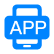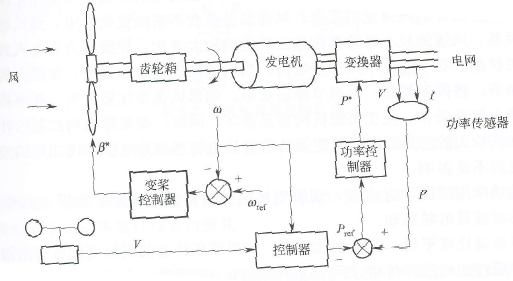APP下载(

)

# 53.某电炉的电阻R为220Ω,接在电压U为220V的电源上,求1h内电炉所放出的热量Q是多少?解:I=U/R=220/220=1( )Q=0.24I2Rt=0.24×12×220×3600=190080( )×4.2=798.34( )10.简述雷击造成叶片损坏的机理?

74.清简述GW82/1500风力发电机组发电机开关柜中过流保护模块的功能?

3个变桨电机的温度

1.简述风力发电机组的低电压穿越要求?

34.双馈异步发单机超同步工作原理是什么?

70.使用摇表测量高压设备绝缘的安全注意事项是什么? (10分)

18.偏航系统的常见故障有哪些?原因是什么?

220/U2=500/475
U2=220×475/500=209( )
P=UI
P2=209×71.8=15006( )

66.简述GW82/1500风力发电机组在额定风速以上和额定风速以下的机组控制方式?

62.根据下图简要说明风电机组变桨距控制原理?# 53.某电炉的电阻R为220Ω,接在电压U为220V的电源上,求1h内电炉所放出的热量Q是多少?解:I=U/R=220/220=1( )Q=0.24I2Rt=0.24×12×220×3600=190080( )×4.2=798.34( )10.简述雷击造成叶片损坏的机理?

74.清简述GW82/1500风力发电机组发电机开关柜中过流保护模块的功能?

3个变桨电机的温度

1.简述风力发电机组的低电压穿越要求?

34.双馈异步发单机超同步工作原理是什么?

70.使用摇表测量高压设备绝缘的安全注意事项是什么? (10分)

18.偏航系统的常见故障有哪些?原因是什么?

220/U2=500/475
U2=220×475/500=209( )
P=UI
P2=209×71.8=15006( )

66.简述GW82/1500风力发电机组在额定风速以上和额定风速以下的机组控制方式?

62.根据下图简要说明风电机组变桨距控制原理?